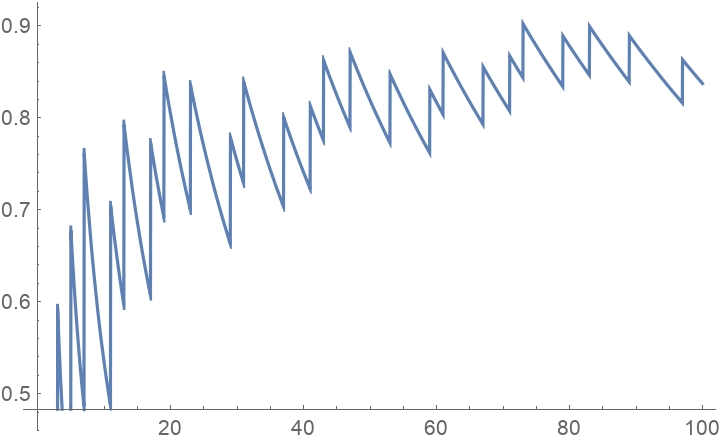#Function Repository Resource:

# ChebyshevTheta

Calculate the value of the first Chebyshev function θ(x)

Contributed by: Jack Heimrath
 ResourceFunction["ChebyshevTheta"][x] returns the value of the first Chebyshev function at x.

## Details and Options

The first Chebyshev function θ(x) is equal to the sum of the logarithms of prime numbers less than or equal to x. For x<2 the sum is empty and by convention we set θ(x)=0.
The Prime Number Theorem is equivalent to the statement that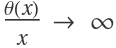as x.
θ(x)=ψ(x)+O(1), where ψ is the second Chebyshev function.

## Examples

### Basic Examples

Compute θ(30):

 In:=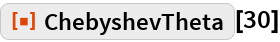Out=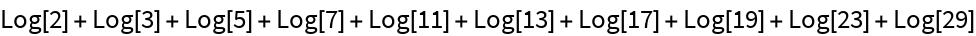Obtain a decimal approximation:

 In:=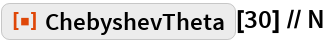Out=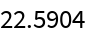Plot θ(x) from 0 to 30:

 In:=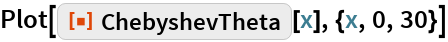Out=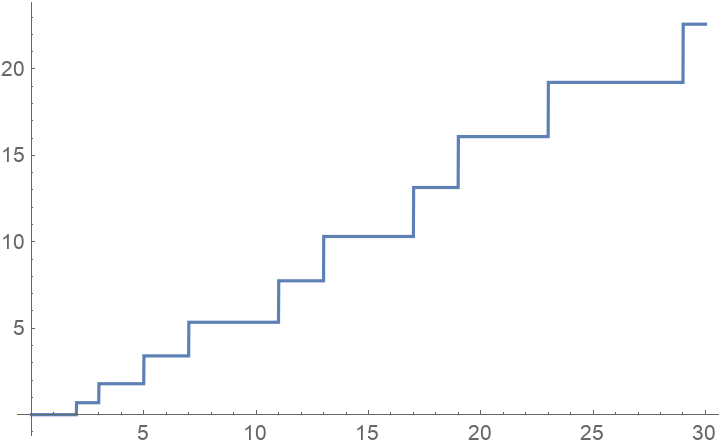Compare x, θ(x), and |x-θ(x)|:

 In:=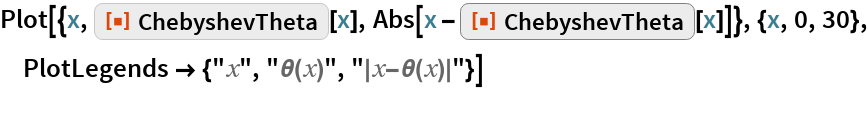Out=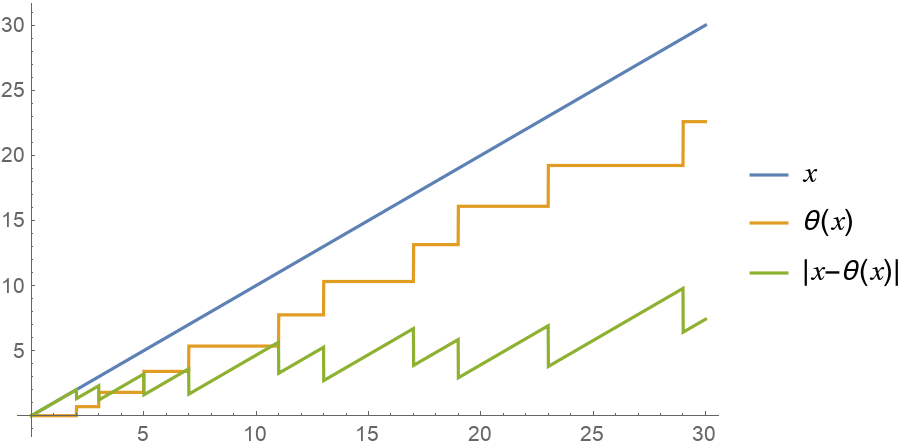Plot the ratio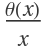:

 In:=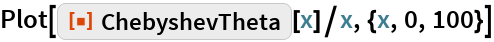Out=